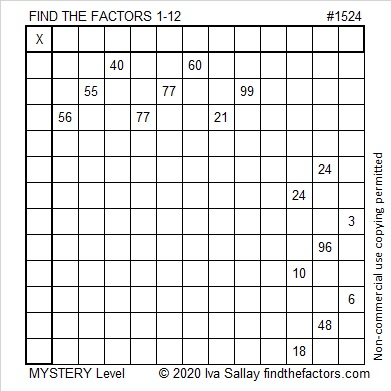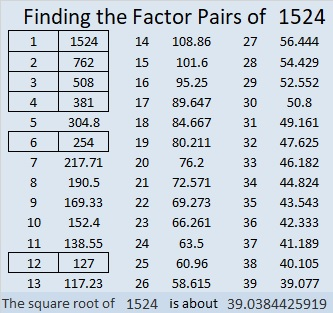# 1524 Mystery

Contents

### Today’s Puzzle:

Is this puzzle easy or difficult? That is the mystery. Will any of the clues trick you into writing factors that won’t work with the rest of the puzzle? If you consistently use logic and not just multiplication and division facts, you’ll solve this puzzle.### Factors of 1524:

• 1524 is a composite number.
• Prime factorization: 1524 = 2 × 2 × 3 × 127, which can be written 1524 = 2² × 3 × 127.
• 1524 has at least one exponent greater than 1 in its prime factorization so √1524 can be simplified. Taking the factor pair from the factor pair table below with the largest square number factor, we get √1524 = (√4)(√381) = 2√381.
• The exponents in the prime factorization are 2, 1, and 1. Adding one to each exponent and multiplying we get (2 + 1)(1 + 1)(1 + 1) = 3 × 2 × 2 = 12. Therefore 1524 has exactly 12 factors.
• The factors of 1524 are outlined with their factor pair partners in the graphic below.### More about the Number 1524:

1524 is the difference of two squares in two different ways:
382² – 380² = 1524,
130² – 124² = 1524.

This site uses Akismet to reduce spam. Learn how your comment data is processed.